• ## 拉格朗日中值定理

千次阅读 2018-04-10 09:40:03
背景：拉格朗日中值定理又称拉氏定理，是罗尔中值定理的推广，同时也是柯西中值定理的特殊情形。法国数学家拉格朗日于1778年在其着作《解析函数论》的第六章提出了该定理，并进行了初步证明，因此人们将该定理命名为...
背景：拉格朗日中值定理又称拉氏定理，是罗尔中值定理的推广，同时也是柯西中值定理的特殊情形。法国数学家拉格朗日于1778年在其着作《解析函数论》的第六章提出了该定理，并进行了初步证明，因此人们将该定理命名为拉格朗日中值定理。配置图片：（转载来自百度）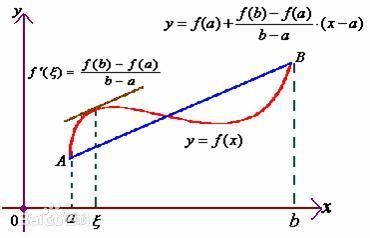概念：如果函数f(x)在(a，b)上可导，[a,b]上连续，则必有一ξ∈(a,b)，使得f'(ξ)*(b-a)=f(b)-f(a)几何意义：若连续曲线y=f(x)在A(a,f(a)),B(b,f(b))两点间的每一点处都有不垂直于x轴的切线,则曲线在A,B间至少存在1点P(c,f(c)),使得该曲线在P点的切线与割线AB平行。物理意义：对于直线运动，在任意一个运动过程中至少存在一个位置（或一个时刻）的瞬时速度等于这个过程中的平均速度。用途：主要用途就是求出函数在一段连续区间上的点的平均值，也就是可以求出函数的最大和最小之间的一个均值。
展开全文• 拉格朗日中值定理求极限 拉格朗日中值定理在理论分析与证题中的重要作用人所共知, 本文通过若干范例说明拉格朗日中值定理也是求某些较难极限的 一种十分简便而有效的工具。数学
• 罗尔定理、拉格朗日中值定理、柯西中值定理罗尔定理拉格朗日中值定理柯西中值定理 罗尔定理 如果一个处处可导的函数的图像和一条水平直线交于不同的两点（图中蓝色两点）， 那么在这两点间的函数图像上至少存在一点...
罗尔定理、拉格朗日中值定理、柯西中值定理罗尔定理拉格朗日中值定理柯西中值定理
罗尔定理
如果一个处处可导的函数的图像和一条水平直线交于不同的两点（图中蓝色两点），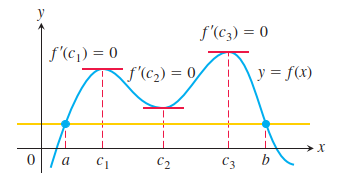那么在这两点间的函数图像上至少存在一点处的切线平行于该水平直线（显然也平行于x轴），
这种现象可以更严谨地表述为罗尔定理：
如果函数f(x)在[a,b]上连续，(a,b) 上可导，并且f(a)=f(b)，那么至少存在一点c于(a,b)内使得$f^{'}\left( c \right) = 0$
罗尔定理的证明要求的是关于导数等于0的结论，我想到的是：
（1）如果f(x)是常数函数的话，那么定义域内任意一点的导数都为0；
（2）可导的函数在极值点处导数为0。所以这里证明的难点是：如果f(x)不是常数函数，那么该怎么证明其有极值存在于(a,b)内呢？若能证明之，则罗尔定理得证。
如果f(x)不是常数函数，因为f(x)在[a,b]上连续，那么在该区间上面必然存在极大值和极小值，假设极大值和极小值均在端点处取得，再加上本定理的条件已经声明f(x)在两端点处的值相等（即f(a)=f(b)），可得出这种情况下函数的极大值等于极小值，这样的函数显然是常数函数，这与开头的假设“f(x)不是常数函数”相悖，所以f(x)不是常数函数情况下其极大值和极小值不可能都在端点处取得——至少存在一个极值点于(a,b)内，又因为f(x)在 (a,b) 上可导，所以该处函数导数为0。
下面是我的证明过程：因为f(x)在[a,b]上连续，那么在该区间上面必然存在极大值和极小值。其极值的分布情况只有两种可能：
（1）若f(x)的极值至少有一个在(a,b)内取得，设该极值点的横坐标为c，因为f(x)在 (a,b) 上可导，所以有$f^{'}\left( c \right) = 0$；
（2）若f(x)的极值均不在(a,b)内取得——极值均在端点处取得，这两个极值分别是f(a)和f(b)，由于本定理的条件中已经声明f(x)在两端点处的值相等（即f(a)=f(b)），可知函数的极大值等于极小值，这样的函数显然是常数函数，那么于(a,b)内的任何一点c都有$f^{'}\left( c \right) = 0$。
综上，至少存在一点c于(a,b)内使得$f^{'}\left( c \right) = 0$，罗尔定理得证
罗尔定理要求 函数f(x)在[a,b]上连续，(a,b) 上可导，这两个条件总让我感觉有些憋扭，因为f(x)在 (a,b) 上可导的话就一定可得出f(x)在 (a,b) 上连续，于是可把条件转化为“函数f(x)在(a,b) 上可导，在a、b两点处连续”，但感觉还是不够简洁，为什么不直接把条件简单地限制为“函数f(x)在[a,b]上可导”呢？
在这个条件下一定会有“函数f(x)在[a,b]上连续，(a,b) 上可导”，后来我想到不做这种简化的原因可能是：函数在a、b两端点处的导数可能是+∞或-∞——不可导，在这种情况下如果把“函数f(x)在[a,b]上连续，(a,b) 上可导”简化成“函数f(x)在[a,b]上可导”就会使罗尔定理不适用于下面这种情况（该函数在-1和1处不可导）：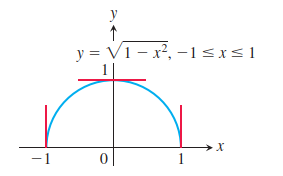认识到这种情形之后，我们可以有一个适用范围小一点但同时也更简洁的罗尔定理：如果函数f(x)在[a,b]上可导，并且f(a)=f(b)，那么至少存在一点c于(a,b)内使得$f^{'}\left( c \right) = 0$。
如果函数f(x)在[a,b]上不连续，那么罗尔定理可能不成立，如图所示：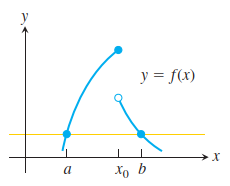如果函数f(x)在(a,b)上不可导，那么罗尔定理可能不成立，如图所示：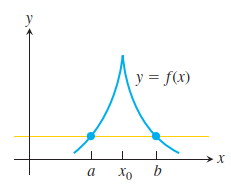上面两图意在让各位认识到罗尔定理的成立条件的必要性。
拉格朗日中值定理
若一条直线和处处可导的函数f(x)的图像交于(a,f(a))和(b,f(b))两点，将该直线上下平移，那么总存在该直线和函数f(x)的图像相切的情形，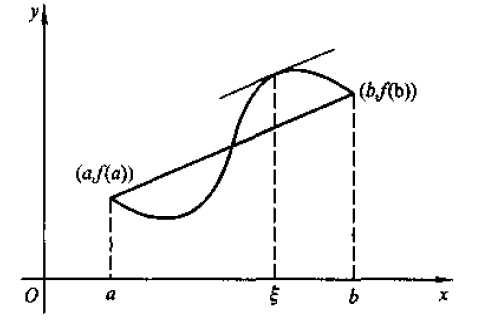这种现象可以更严谨地表述为微分中值定理（亦称拉格朗日中值定理）：如果函数f(x)在[a,b]上连续，(a,b) 上可导，那么至少存在一点c于(a,b)内使得$f^{'}\left( c \right) = \frac{f(b) - \ f(a)}{b - a}$
微分中值定理可以看作是罗尔定理旋转后的情形,可设想把满足罗尔定理的图像旋转一个角度后，那么原来过(a,f(a))和(b,f(b))的水平直线变成了斜率为$\frac{f(b) - \ f(a)}{b - a}$的直线，而那条切线始终与之平行，所以斜率（该点的导数）依然等于$\frac{f(b) - \ f(a)}{b - a}$
微分中值定理也可以用罗尔定理来证明，如下：
过(a,f(a))和(b,f(b))的直线的方程是$g\left( x \right) = f\left( a \right) + \frac{f\left( b \right) - \ f\left( a \right)}{b - a}(x - a)$，f(x)和g(x)的纵向差距可表示为$h\left( x \right) = f\left( x \right) - g\left( x \right) = f\left( x \right) - f\left( a \right) - \frac{f\left( b \right) - \ f\left( a \right)}{b - a}(x - a)$，因为f(x)和g(x)的图像在两端点处相交，所以h(a)=h(b)=0，同时不难得出h(x)在[a,b]上连续，(a,b) 上可导，所以h(x)满足罗尔定理，因而存在一点c于(a,b)内使得$h'(c) = 0 = f'\left( c \right) - \frac{f\left( b \right) - \ f\left( a \right)}{b - a}$，进而可得出$f^{'}(c) = \frac{f\left( b \right) - \ f\left( a \right)}{b - a}$，微分中值定理得证。
作为微分中值定理的应用，我们可以考虑这样一种情形：假如一辆车做变速运动，一小时行了20km，如果f(x)是车的位移函数、f(0)=0、f(1)=20，微分中值定理告诉我们在这一小时内必然有一刻车速为$\frac{f\left( 1 \right) - \ f\left( 0 \right)}{1 - 0} = \frac{20 - 0}{1 - 0} = 20(km/h)$，如果你对此仍怀疑，那么请设想其反面：若这一小时内车速始终大于或小于20km/h会出现什么情况？……所以这一小时内车速绝对会有一刻为20km/h。
对比一下微分中值定理和罗尔定理的差异，我们不难发现微分中值定理可以囊括罗尔定理的情形——微分中值定理中f(a)=f(b)的时候它便退化成了罗尔定理，也就是说微分中值定理具有更普遍的适用范围。
柯西中值定理
现在让我们来看一个更广义的微分中值定理（亦称柯西中值定理）：如果f(x)和g(x)都在[a,b]上连续，(a,b) 上可导，如果在(a,b)上g′(x)≠0，那么至少存在一点c于(a,b)内使得
$\frac{f'(c)}{g^{'}\left( c \right)} = \frac{f(b) - f(a)}{g\left( b \right) - g\left( a \right)}$
为什么说该定理是更广义的微分中值定理呢？微分中值定理就是上面这个等式中令g(x)=x的情形。至于广义微分中值定理的证明，我们只用令$h\left( x \right) = \left\lbrack f\left( b \right) - f\left( a \right) \right\rbrack g\left( x \right) - \left\lbrack g\left( b \right) - g\left( a \right) \right\rbrack f\left( x \right)$，
然后对其应用微分中值定理便不难得证
参考自：https://www.cnblogs.com/iMath/p/10158670.html


展开全文• 拉格朗日中值定理的内容、几何意义和证明过程的讲解
• 下面实战演练拉格朗日中值定理的证明。 先给出拉格朗日中值定理的定义： 如果函数f(x)满足： （1）在闭区间[a,b]上连续； （2）在开区间(a,b)内可导； 那么在开区间(a,b)内至少有一点   作者：披风秃头侠
• 1.罗尔中值定理 ...2.拉格朗日中值定理（微分中值定理/罗尔中值定理的推广） 3.柯西中值定理（拉格朗日总值定理的推广） 参考文章：https://blog.csdn.net/FnqTyr45/article/details/80037454 ...
1.罗尔中值定理
如果一个处处可导的函数的图像和一条水平直线交于不同的两点（如图所示），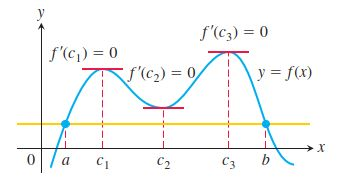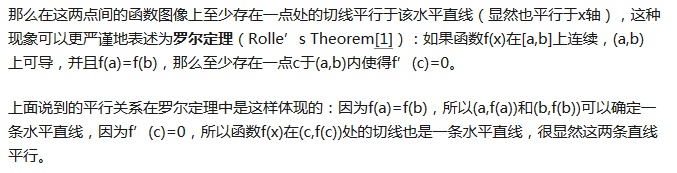2.拉格朗日中值定理（微分中值定理/罗尔中值定理的推广）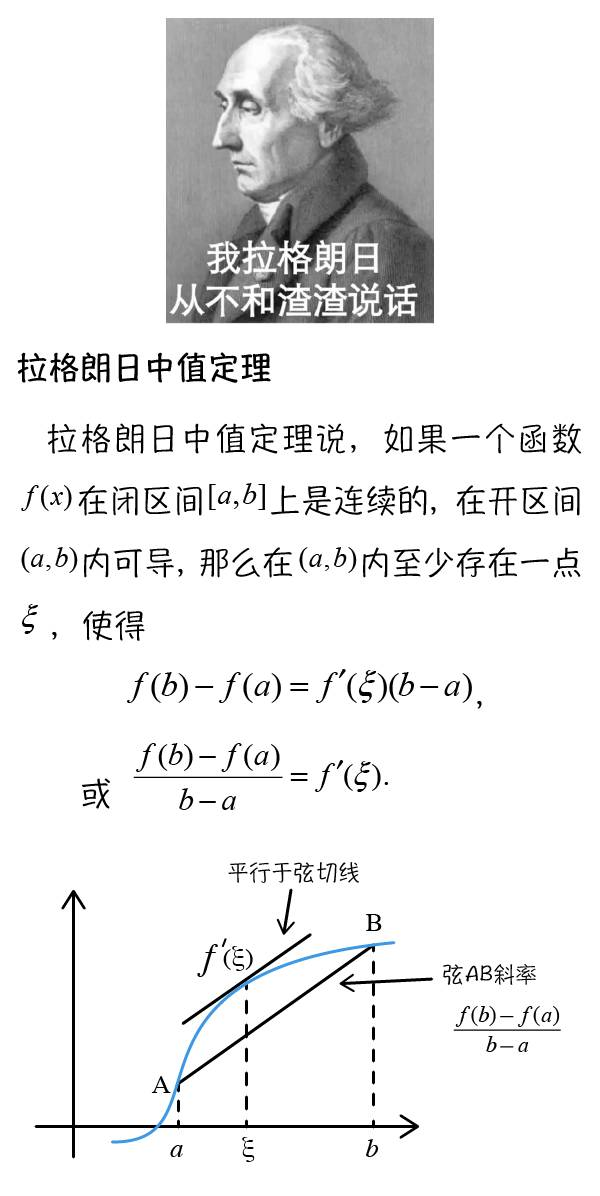3.柯西中值定理（拉格朗日总值定理的推广）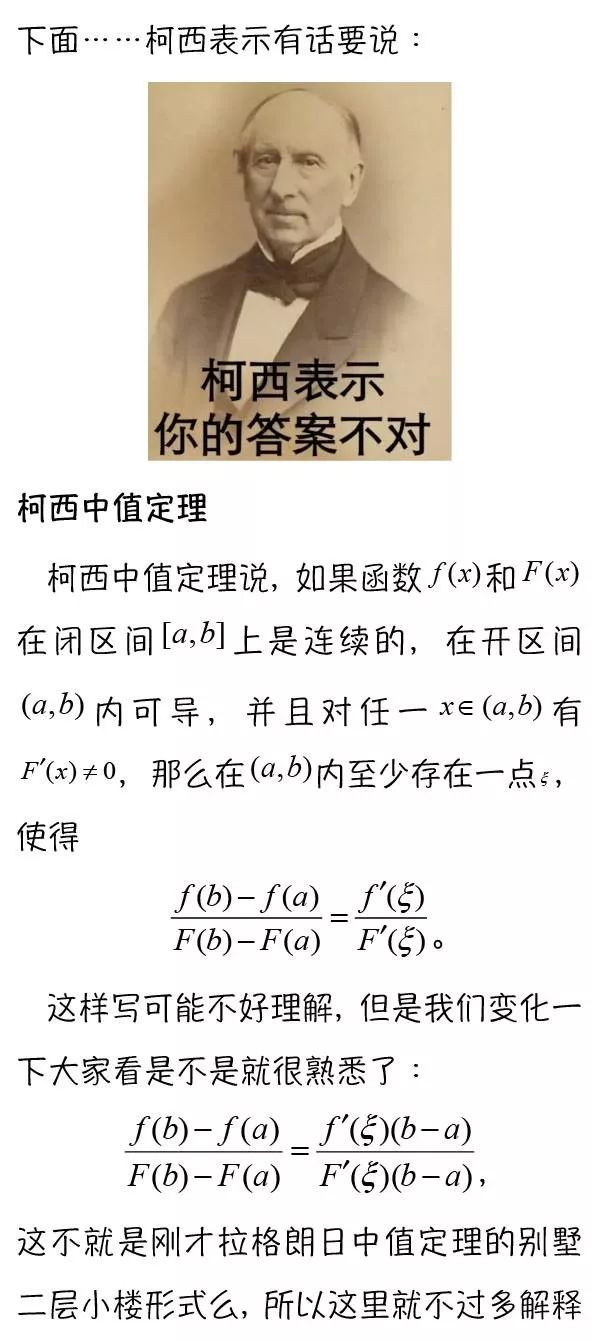参考文章：https://blog.csdn.net/FnqTyr45/article/details/80037454


展开全文• 罗尔定理证明；拉格朗日中值定理证明；柯西中值定理证明； 证明方法挑选的基本都是步骤简单的。

高等数学的学习躲不过中值定理，而这部分内容又是有些难度，由于检索相关三大微分中值定理定理的证明并没有满意的文章，便自己整理了一篇供自己参考，希望也能为各位读者提供一些帮助！

1 罗尔定理
描述
如果 R 上的函数 f(x) 满足以下条件：（1）在闭区间 [a,b] 上连续，（2）在开区间 (a,b) 内可导，（3）f(a)=f(b)，则至少存在一个 ξ∈(a,b)，使得 f’(ξ)=0。
证明
因为函数 f(x) 在闭区间[a,b] 上连续，所以存在最大值与最小值，分别用M 和 m 表示，分两种情况讨论：

若 M=m，则函数 f(x) 在闭区间 [a,b] 上必为常函数，结论显然成立。

若 M>m，则因为 f(a)=f(b) 使得最大值 M 与最小值 m 至少有一个在 (a,b) 内某点ξ处取得，从而ξ是f(x)的极值点，
又条件 f(x) 在开区间 (a,b) 内可导得，f(x) 在 ξ 处取得极值，由费马引理推知：f’(ξ)=0。助解

费马引理，总结就一句话：可导函数极值点为零
若M>m的情况借助图像，便于理解

2 拉格朗日中值定理
描述证明注：证法不唯一
通过一段时间的中值定理相关证明题的学习，不难发现辅助函数的构造在解题中的重要性。
3 柯西中值定理参考链接
1. 知乎拉格朗日定理证明
2. 百度知道


展开全文高等数学 罗尔定理 柯西中值定理
• 拉格朗日中值定理 积分中值定理 导数定义： 拉格朗日中值定理 如果函数f(x)满足： （1）在闭区间[a,b]上连续； （2）在开区间(a,b)内可导； 那么在开区间(a,b)内至少有一点 积分中值定理 导数定义： ...
• 拉格朗日中值定理拉格朗日中值定理说，如果一个函数f(x)在闭区间[a,b]上是连续的，在开区间(a,b)内可导，那么在(a,b)内至少存在一点ξ，使得或拉格朗日中值定理的意思就是：连接图像上两个点 A、B画一条线，要求画...
• 高数篇：04拉格朗日中值定理高数篇：04拉格朗日中值定理定理7：拉格朗日中值定理拉格朗日的应用拉格朗日与罗尔定理的区别转载需注明出处 高数篇：04拉格朗日中值定理 定理7：拉格朗日中值定理 下面提出拉格朗日中值...
• 对于史济怀老师证明拉格朗日中值定理的方法感觉太突兀了，在看知乎关于史济怀老师那本书的评论时，甚至有人因为一个定理的证明就否定整本书，我觉得还是欠妥，我个人认为，史济怀老师的书以及讲课视频还是挺不错的。...
• 拉格朗日中值定理 主条目：拉格朗日中值定理 拉格朗日中值定理的几何意义 如果函数f(x)满足 在闭区间[a,b]上连续；在开区间(a,b)内可导， 那么在(a,b)内至少有一点ξ(a b)，使等式 成立...
• 拉格朗日中值定理的定义：如果函数f(x)在[a,b]上连续，且在(a,b)可导，则函数f(x)上必有一点p，使得：(f(b)-f(a) )/(b-a)=f'(p)。该定理可以认为如果函数满足拉格朗日中值定理所有条件，那么f(x)上两点连线构成的...
• 3.1中值定理1. 费马定理设函数在点的某邻域内有定义并且在处可导， 如果对任意的，有(或),那么2. 罗尔定理如果函数在闭区间上连续,在开区间内可导,且在... 拉格朗日中值定理(微分中值定理)如果函数在闭区间上连续...
• 摘要： 利用拉格朗日中值定理可以解决一类极限问题，此法关键之处在于找到相应的函数和相应的自变量，本文通过几个具体事例分析来帮助大家更好的理解应用！拉格朗日中值定理回顾若函数满足如下条件：(ⅰ)在闭区间上...
• Lagrange’s Mean Value Theorem - 拉格朗日中值定理 Lagrange [lə'ɡrɑndʒ]：n. 拉格朗日 (法国著名数学家，力学家) 拉格朗日中值定理又称为拉氏定理，它反映了可导函数在闭区间上的整体的平均变化率与区间内某...
• ## 利用拉格朗日中值定理求极限

千次阅读 多人点赞 2020-03-17 11:47:12
对有一些形式，使用拉格朗日中值定理非常便捷。下面举两个个例子： 这种形式的式子，很明显直接使用等价无穷小是不行的，洛必达法则又麻烦至极，泰勒公式做起来也不轻松。 我们发现上述式子有这样的特点：右侧...
• 证明过程：【2】罗尔定理的证明证明思路：1、用费马定理来证明2、题目没有更多要求的话也可以用拉格朗日中值定理秒杀(不讲武德法，略去)证明过程：【3】拉格朗日中值定理证明证明思路：造辅助函数，然后用罗...
• 下面这个图像不错，例1可以，说明只要是在开区间内求导为0，就一定是常数。...罗尔定理和拉格朗日中值定理 我们都把它们称为 微分中值定理，微分中值定理是建立函数与导数的桥梁。这句话 ，重在体会不需要理解。 ...算法
• 1. 问题引入——罚单合理性问题 ...4. 拉格朗日中值定理（若函数在闭区间上连续，在开区间内可导，则至少存在一点其导数可表示为端点上的函数值之差与自变量的增量之比） 5. 拉格朗日中值定理的其他形式 ...
• 拉格朗日中值定理又称拉氏定理，是微分学中的基本定理之一，它反映了可导函数在闭区间上的整体的平均变化率与区间内某点的局部变化率的关系。
• 拉格朗日中值定理 https://www.bilibili.com/video/BV117411E7kx?from=search&seid=17921778669593975548 拉格朗日中值定理的证明一直是让同学们头疼的一个问题，往往教科书仅仅给出辅助函数，但不做过多的解释...
• 拉格朗日中值定理 必定存在 a&amp;lt;ξ&amp;lt;b, 令 f’(ξ) = ( f(b) - f(a) ) / (b - a) 柯西中值定理 必定存在 a&amp;lt;ξ&amp;lt;b, 令 f’(ξ) / F’(ξ) = ( f(b) - f(a.....